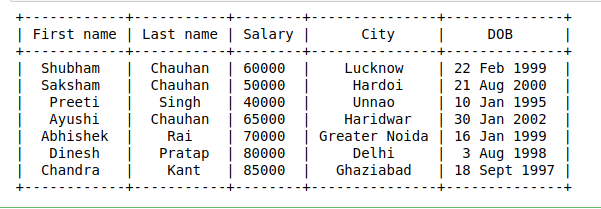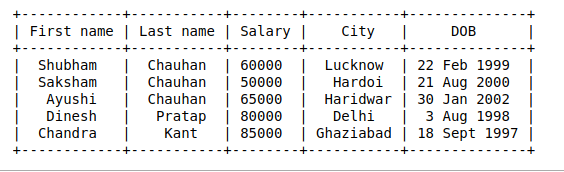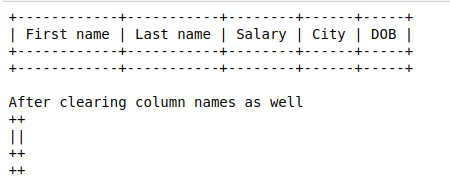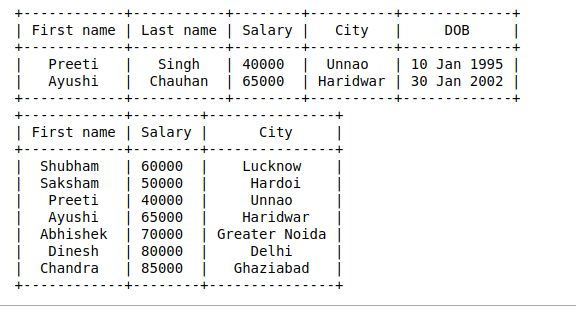Open in App
Not now

# Generate simple ASCII tables using prettytable in Python

• Last Updated : 29 Aug, 2020

Prettytable is a Python library used to print ASCII tables in an attractive form and to read data from CSV, HTML, or database cursor and output data in ASCII or HTML. We can control many aspects of a table, such as the width of the column padding, the alignment of text, or the table border.

### Installation

In order to be able to use prettytable library first we need to
install it using pip tool command:

```pip install prettytable
```

## Inserting data

Data can be inserted in a PrettyTable either row by row or column by column.

### Inserting data row by row

To do this you can set the field names first using the field_names attribute, and then add the rows one at a time using the add_row method.
Example:

## Python3

 `# importing required library``from` `prettytable ``import` `PrettyTable`` ` `# creating an empty PrettyTable``x ``=` `PrettyTable()`` ` `# adding data into the table``# row by row``x.field_names ``=` `[``"First name"``, ``"Last name"``, ``"Salary"``, ``"City"``, ``"DOB"``]``x.add_row([``"Shubham"``, ``"Chauhan"``, ``60000``, ``"Lucknow"``, ``"22 Feb 1999"``])``x.add_row([``"Saksham"``, ``"Chauhan"``, ``50000``, ``"Hardoi"``, ``"21 Aug 2000"``])``x.add_row([``"Preeti"``, ``"Singh"``, ``40000``, ``"Unnao"``, ``"10 Jan 1995"``])``x.add_row([``"Ayushi"``, ``"Chauhan"``, ``65000``, ``"Haridwar"``, ``"30 Jan 2002"``])``x.add_row([``"Abhishek"``, ``"Rai"``, ``70000``, ``"Greater Noida"``, ``"16 Jan 1999"``])``x.add_row([``"Dinesh"``, ``"Pratap"``, ``80000``, ``"Delhi"``, ``"3 Aug 1998"``])``x.add_row([``"Chandra"``, ``"Kant"``, ``85000``, ``"Ghaziabad"``, ``"18 Sept 1997"``])`` ` `# printing generated table``print``(x)`

Output:### Inserting data column by column

To do this you use the add_column method, which takes two arguments – a string which is the name for the field the column you are adding corresponds to, and a list or tuple which contains the column data”
Example:

## Python3

 `# importing required library``from` `prettytable ``import` `PrettyTable`` ` `# creating an empty PrettyTable``x ``=` `PrettyTable()`` ` `# adding data into the table``# column by column``x.add_column(``"First name"``,``             ``[``"Shubham"``, ``"Saksham"``, ``"Preeti"``, ``"Ayushi"``,``              ``"Abhishek"``, ``"Dinesh"``, ``"Chandra"``])`` ` `x.add_column(``"Last name"``, [``"Chauhan"``, ``"Chauhan"``, ``"Singh"``,``                           ``"Chauhan"``, ``"Rai"``, ``"Pratap"``,``                           ``"Kant"``])`` ` `x.add_column(``"Salary"``, [``60000``, ``50000``, ``40000``, ``65000``, ``70000``,``                        ``80000``, ``85000``])``x.add_column(``"City"``, [``"Lucknow"``, ``"Hardoi"``, ``"Unnao"``, ``"Haridwar"``,``                      ``"Greater Noida"``, ``"Delhi"``, ``"Ghaziabad"``])`` ` `x.add_column(``"DOB"``, [``"22 Feb 1999"``, ``"21 Aug 2000"``, ``"10 Jan 1995"``,``                     ``"30 Jan 2002"``, ``"16 Jan 1999"``, ``"3 Aug 1998"``,``                     ``"18 Sept 1997"``])`` ` `# printing generated table``print``(x)`

Output:## Deleting Data

A specific row can be deleted using the del_row() method. Index of the row to be deleted is passed as a parameter.

Example:

## Python3

 `# importing required library``from` `prettytable ``import` `PrettyTable`` ` `# creating an empty PrettyTable``x ``=` `PrettyTable()`` ` `# adding data into the table``# column by column``x.add_column(``"First name"``,``             ``[``"Shubham"``, ``"Saksham"``, ``"Preeti"``, ``"Ayushi"``,``              ``"Abhishek"``, ``"Dinesh"``, ``"Chandra"``])`` ` `x.add_column(``"Last name"``, [``"Chauhan"``, ``"Chauhan"``, ``"Singh"``,``                           ``"Chauhan"``, ``"Rai"``, ``"Pratap"``,``                           ``"Kant"``])`` ` `x.add_column(``"Salary"``, [``60000``, ``50000``, ``40000``, ``65000``, ``70000``,``                        ``80000``, ``85000``])``x.add_column(``"City"``, [``"Lucknow"``, ``"Hardoi"``, ``"Unnao"``, ``"Haridwar"``,``                      ``"Greater Noida"``, ``"Delhi"``, ``"Ghaziabad"``])`` ` `x.add_column(``"DOB"``, [``"22 Feb 1999"``, ``"21 Aug 2000"``, ``"10 Jan 1995"``,``                     ``"30 Jan 2002"``, ``"16 Jan 1999"``, ``"3 Aug 1998"``,``                     ``"18 Sept 1997"``])`` ` `# Deleting row``x.del_row(``2``)``x.del_row(``3``)`` ` `# printing generated table``print``(x)`

Output:All the rows in the table can be deleted using the clear_rows() method. This method will keep the column names. To delete both rows and columns name clear() method is used.

Example:

## Python3

 `# importing required library``from` `prettytable ``import` `PrettyTable`` ` `# creating an empty PrettyTable``x ``=` `PrettyTable()`` ` `# adding data into the table``# column by column``x.add_column(``"First name"``,``             ``[``"Shubham"``, ``"Saksham"``, ``"Preeti"``, ``"Ayushi"``,``              ``"Abhishek"``, ``"Dinesh"``, ``"Chandra"``])`` ` `x.add_column(``"Last name"``, [``"Chauhan"``, ``"Chauhan"``, ``"Singh"``,``                           ``"Chauhan"``, ``"Rai"``, ``"Pratap"``,``                           ``"Kant"``])`` ` `x.add_column(``"Salary"``, [``60000``, ``50000``, ``40000``, ``65000``, ``70000``,``                        ``80000``, ``85000``])``x.add_column(``"City"``, [``"Lucknow"``, ``"Hardoi"``, ``"Unnao"``, ``"Haridwar"``,``                      ``"Greater Noida"``, ``"Delhi"``, ``"Ghaziabad"``])`` ` `x.add_column(``"DOB"``, [``"22 Feb 1999"``, ``"21 Aug 2000"``, ``"10 Jan 1995"``,``                     ``"30 Jan 2002"``, ``"16 Jan 1999"``, ``"3 Aug 1998"``,``                     ``"18 Sept 1997"``])`` ` `# Deleting all rows``x.clear_rows()`` ` `print``(x)`` ` `# Deleting column name as well``print``(``"\nAfter clearing column names as well"``)``x.clear()`` ` `print``(x)`

Output:## Controlling which data gets displayed

You can control which rows or which columns are going to be displayed.

## Python3

 `# importing required library``from` `prettytable ``import` `PrettyTable`` ` `# creating an empty PrettyTable``x ``=` `PrettyTable()`` ` `# adding data into the table``# column by column``x.field_names ``=` `[``"First name"``, ``"Last name"``, ``"Salary"``, ``"City"``, ``"DOB"``]``x.add_row([``"Shubham"``, ``"Chauhan"``, ``60000``, ``"Lucknow"``, ``"22 Feb 1999"``])``x.add_row([``"Saksham"``, ``"Chauhan"``, ``50000``, ``"Hardoi"``, ``"21 Aug 2000"``])``x.add_row([``"Preeti"``, ``"Singh"``, ``40000``, ``"Unnao"``, ``"10 Jan 1995"``])``x.add_row([``"Ayushi"``, ``"Chauhan"``, ``65000``, ``"Haridwar"``, ``"30 Jan 2002"``])``x.add_row([``"Abhishek"``, ``"Rai"``, ``70000``, ``"Greater Noida"``, ``"16 Jan 1999"``])``x.add_row([``"Dinesh"``, ``"Pratap"``, ``80000``, ``"Delhi"``, ``"3 Aug 1998"``])``x.add_row([``"Chandra"``, ``"Kant"``, ``85000``, ``"Ghaziabad"``, ``"18 Sept 1997"``])`` ` `# With the start and end parameters, we can select``# which rows to display in the output.``print``(x.get_string(start``=``2``, end``=``4``))`` ` `# With the fields option we can select columns``# which are going to be displayed.``print``(x.get_string(fields``=``[``"First name"``, ``"Salary"``, ``"City"``]))`

Output:### Various other operations which can be performed

• Sorting(ascending or descending) can be performed using the sortby property, in which we sort the table by specifying a column to be sorted.
• HTML output of the table can be generated using the get_html_string method.

## Python3

 `# importing required library``from` `prettytable ``import` `PrettyTable`` ` `# creating an empty PrettyTable``x ``=` `PrettyTable()`` ` `# adding data into the table``# row by row``x.field_names ``=` `[``"First name"``, ``"Last name"``, ``"Salary"``, ``"City"``, ``"DOB"``]``x.add_row([``"Shubham"``, ``"Chauhan"``, ``60000``, ``"Lucknow"``, ``"22 Feb 1999"``])``x.add_row([``"Saksham"``, ``"Chauhan"``, ``50000``, ``"Hardoi"``, ``"21 Aug 2000"``])``x.add_row([``"Preeti"``, ``"Singh"``, ``40000``, ``"Unnao"``, ``"10 Jan 1995"``])``x.add_row([``"Ayushi"``, ``"Chauhan"``, ``65000``, ``"Haridwar"``, ``"30 Jan 2002"``])``x.add_row([``"Abhishek"``, ``"Rai"``, ``70000``, ``"Greater Noida"``, ``"16 Jan 1999"``])``x.add_row([``"Dinesh"``, ``"Pratap"``, ``80000``, ``"Delhi"``, ``"3 Aug 1998"``])``x.add_row([``"Chandra"``, ``"Kant"``, ``85000``, ``"Ghaziabad"``, ``"18 Sept 1997"``])`` ` `# printing generated table``print``(``"Original Table:"``)``print``(x)`` ` `# printing the HTML string of this table``print``(``"\nHTML code for this Table:"``)``print``(x.get_html_string())`` ` `# printing table after sorting(ascending) ``# by column salary``x.sortby ``=` `"Salary"``print``(``"\nSorted Table by Salary:"``)``print``(x.get_string())`` ` `# printing table after sorting(ascending) ``# by column city``x.sortby ``=` `"City"``x.reversesort ``=` `True``print``(``"\nReverse Sorted Table by City:"``)``print``(x.get_string())`

Output:

My Personal Notes arrow_drop_up